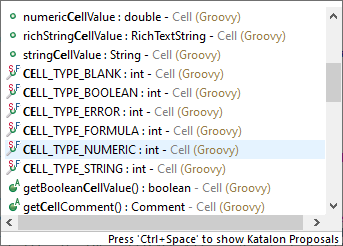# How to get data from excel as Integer and NOT BigDecimal

Hello friends,

i tried out data-driven testing the first time.
That means I used the “Data Binding” option of my testcases to connect it to data I store in an excel-file (and different sheets).

In one case I have columns that store strings, and numbers. The numbers I need as Integers in my testcase.
In the testcase I call my own method and pass the Strings and Integers I try to get from the excel.
My method expects two Strings and then three Integer values, but it always gets it as BigDecimal from the excel:

``````groovy.lang.MissingMethodException: No signature of method: package.of.my.method.methodName() is applicable for argument types: (java.lang.String, java.lang.String, java.math.BigDecimal, java.math.BigDecimal, java.math.BigDecimal) values: [text, text2, 5.0, 999999999, 3.0]
``````

At first I thought the value 999999999 is too big for Integer, what could have been a reason for using BigDecimal, but the maximum value is 2147483647 which is higher than my value.
But to be sure I tried it again with a smaller value and I still got it as BigDecimal:
[text, text2, 5.0, 999.0, 3.0]

In MS Excel I formated the cells as Text and Numbers (numbers without decimal places).

Is there a way to tell Katalon to get numbers as Integers and not BigDecimal?
Or do I need to convert it on myself everytime bevore I process it?

Thanks & all the best,
Simon

Since we can’t mandate what happens in 3rd party application like MS Excel, it would probably be prudent to try to get numbers as Integers AND convert it yourself as a safety measure.

For MS Excel, there are supposed to be various cell types that you can set, like:At the same time, I would add …(text, text2, 5.0 as int, 999999999 as int, 3.0 as int) to your parameter list.

At which point can you set these cell types? I don’t get what you mean.

I use the “Variables”-tab of my testcase to set the parameter names and types. Then I use the “Data Binding”-tab of the same testcase to connect these variables to my excel-columns.

So the types of these variables are set in this “Variables”-tab.
Inside of my Testcase-Script I can use this names, but at this scope the script does not know anymore that this values come from an an excel-cell. For Katalon this is just a variable of type String or Number.

What I did so far, as I know these Numbers come in as BigDecimal, is the following:

``````BigDecimal decimalVar = variable03;
Integer integerVar = decimalVar.intValue();
``````

But this “as int” keyword looks much shorter. I haven’t seen this before.
Do you place it inside of the method call?

``````object.methodName(variable03 as int)
``````

like this?

Thanks!

or as

`object.methodName((int)variable03)`

Edit: Just a note that I usually get all my values as Strings and then convert to numbers only for any math calculations. Otherwise, numbers are not needed.

``````houseNumber = data.getValue(2, row)   // "52147"
WebUI.setText(findTestObject('myPage/input_GeneralInfo.Street2'),  houseNumber )
``````

Ok, I thought the same, that it is easier to set every parameter to String.
In the excel I formated every cell to “text”. And in my “Variables”-tab of my testcase I also set every variable to String and not number anymore.
But I still get the same MissingMethodException, because it automatically converts some values to BigDecimal.
This is totally annoying